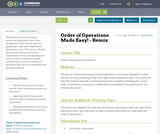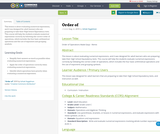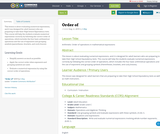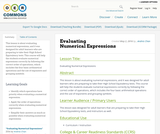# 4 Results

View
Selected filters:
• Grouping Symbols
RemixConditions of Use:
No Strings Attached
Rating

This lesson is about evaluating numerical expressions, and it was designed for adult learners who are preparing to take their High School Equivalency tests. This course will help the students evaluate numerical expressions correctly by following the correct order of operations, which includes the four basic arithmetical operations and the use of exponents and grouping symbols.

Subject:
Mathematics
Material Type:
Lesson
Author:
Susan Jones
08/08/2019
RemixConditions of Use:
No Strings Attached
Rating

This lesson is about evaluating numerical expressions, and it was designed for adult learners who are preparing to take their High School Equivalency tests. This course will help the students evaluate numerical expressions correctly by following the correct order of operations, which includes the four basic arithmetical operations and the use of exponents and grouping symbols.

Subject:
Mathematics
Material Type:
Lesson
Author:
Itzhak Feygelman
08/08/2019
RemixConditions of Use:
No Strings Attached
Rating

This lesson is about evaluating numerical expressions, and it was designed for adult learners who are preparing to take their High School Equivalency tests. This course will help the students evaluate numerical expressions correctly by following the correct order of operations, which includes the four basic arithmetical operations and the use of exponents and grouping symbols (parentheses, brackets, and curly braces).

Subject:
Mathematics
Material Type:
Lesson
Author:
S Roy
08/08/2019Conditions of Use:
No Strings Attached
Rating

This lesson is about evaluating numerical expressions, and it was designed for adult learners who are preparing to take their High School Equivalency tests. This course will help the students evaluate numerical expressions correctly by following the correct order of operations, which includes the four basic arithmetical operations and the use of exponents and grouping symbols.

Subject:
Mathematics
Material Type:
Lesson
Author:
Andres Chan